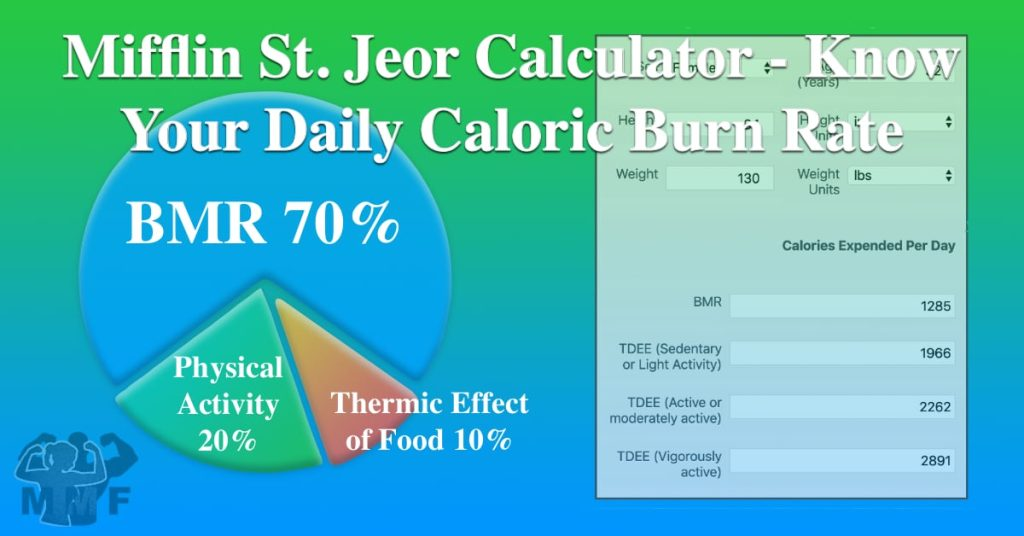# Mifflin St. Jeor Calculator – Know Your Daily Caloric Burn Rate (BMR) (TDEE)Mifflin St. Jeor BMR and TDEE Calculator is a quick way to estimate how many calories you burn per day. If you are trying to lose or gain weight you need to calculate how many calories you use a day.

Your Basal Metabolic Rate (BMR) is the amount of energy you expend daily while at rest. When you multiply your BMR by your activity level you get your Total Energy Expenditure (TDEE).

#### The Harris–Benedict equations revised by Mifflin and St Jeor in 1990 (1).

Sex Calculation
Men BMR = (10 × weight in kg) + (6.25 × height in cm) – (5 × age in years) + 5
Women BMR = (10 × weight in kg) + (6.25 × height in cm) – (5 × age in years) – 161

#### Total Daily Energy Expenditure (TDEE) is BMR multiplied by Physical Activity Level (PAL) (2).

Lifestyle Example PAL Calculation
Sedentary or light activity Office worker getting little or no exercise 1.53 BMR x 1.53
Active or moderately active Construction worker or person running one hour daily 1.76 BMR x 1.76
Vigorously active Agricultural worker (non mechanized) or person swimming two hours daily 2.25 BMR x 2.25

### Understanding Your Total Daily Energy Expenditure

Your TDEE is made up of three parts your BMR, PAL, and Thermic Effect of Food (TEF). We have already discussed how BMR is the amount of energy in Kilocalories that your body uses while at rest. The Above TDEE equation calculates TDEE using a multiplication factor for the PAL. Your PAL can range from very low for a sedentary life to very high for an Olympic swimmer. For the above TDEE equation, the multiple PAL takes into account your TEF as well.

#### Calculating Your Thermic Effect of Food

TEF also known as Dietary Induced Thermogenesis (DIT) or Specific Dynamic Action (SDA) is the amount of energy expenditure above your BMR due to your body processing and storing food. Since every food has a different TEF, calculating TEF becomes very complicated. One helpful piece of information about TEF is that Protein has more than double the TEF of Carbohydrates and Fat. Because TEF is so difficult to calculate it is commonly assumed to be a multiple of the number of calories consumed or amount of calories expended (BMR).

For a more precise measurement of your BMR you can use the Resting Daily Energy Expenditure Calculator Here: Katch-McArdle Calculator – Get Your Resting Daily Energy Expenditure (RDEE)